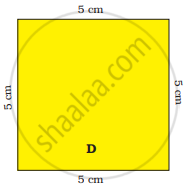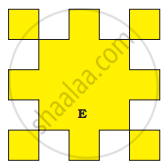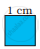# Find the length of the boundary of square D. 8 squares of side 1 cm are cut out of the square D. Now it looks like shape E. What is the length of the b - Mathematics

Sum
1. Find the length of the boundary of square D.
2. 8 squares of side 1 cm are cut out of the square D. Now it looks like shape E. What is the length of the boundary of shape E?3. The boundary of thisis ______ + ______ + ______ + ______
Can we also say that the boundary is 4 × 1 cm?

#### Solution

1. The boundary of shape D = 5 cm + 5 cm + 5 cm + 5 cm = 20 cm
2. There are 21 edges measuring 1 cm of shape ‘E’.
Hence, the boundary of shape ‘E’ = 1 cm x 21 = 21 cm
3. The boundary= 1 cm + 1 cm + 1 cm + 1 cm = 4 cm
So, we can say the boundary of= 4 × 1 cm.
Concept: Fields and Fences
Is there an error in this question or solution?

#### APPEARS IN

NCERT Mathematics - Math - Magic Class 4 CBSE
Chapter 13 Fields and Fences
Q 2. | Page 155